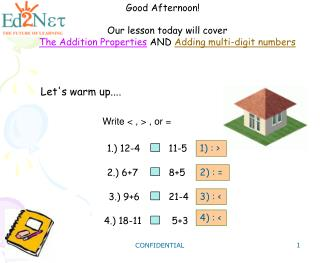DownloadDownload PresentationGood Afternoon!

# Good Afternoon!

Download Presentation## Good Afternoon!

- - - - - - - - - - - - - - - - - - - - - - - - - - - E N D - - - - - - - - - - - - - - - - - - - - - - - - - - -
##### Presentation Transcript

1. Good Afternoon! Our lesson today will cover The Addition Properties AND Adding multi-digit numbers Let's warm up.... Write < , > , or = 1.) 12-4 11-5 1) : > 2.) 6+7 8+5 2) : = 3.) 9+6 21-4 3) : < 4) : < 4.) 18-11 5+3 CONFIDENTIAL

2. Let's review what we covered in the last lesson. We can use PLACE VALUE to ROUND. Let's round 2,345to the nearest thousands place. First, we need to underline the thousands place. 2,345 CONFIDENTIAL

3. 2,345 Second, we need to look at the digit just to the right of the underlined digit. * If that digit is 5 ormore, we increase the underlined digit by 1. * If that digit is 4or less, we do not change the underlined digit. CONFIDENTIAL

4. 2,345 Since this digit is 4 or less, we leave the 2alone. Finally, we changeall the digits to the right of the underlined digit to 0. 2,345 rounds to 2000 CONFIDENTIAL

5. SET of data 52, 45, 48, , 69, 74 The middle number is called the MEDIAN CONFIDENTIAL

6. ADDITION OF MULTI-DIGIT NUMBERS There are several properties of addition COMMUTATIVE PROPERTY: We can change the order and the sum stays the same. EXAMPLE: 4 + 6 = 10 6 + 4 = 10 ASSOCIATIVE PROPERTY: We can change the grouping and the sum stays the same. EXAMPLE: 2 + (3+ 8) = 10 (2 + 3)+ 8= 10 IDENTITY PROPERTY: We can add zero and the sum stays the same. EXAMPLE: 7 +0 = 0 CONFIDENTIAL

7. Complete each problem using the COMMUTATIVE PROPERTY. "Change the Order" 36 + 98 = 98 + 36 + + 0 + 76 = 76 + 0 CONFIDENTIAL

8. Complete each problem using the ASSOCIATIVE PROPERTY. "Re-group" 20 + (4 + 6) = ( (20 + 4) + 6 + ) + (8 + 7) + 5 = ) ( + + 8 + (7 + 5) CONFIDENTIAL

9. Complete each problem using the IDENTITY PROPERTY. "Keep the same number" 15 15 + 0 = + 34 = 34 0 CONFIDENTIAL

10. We add numbers together by first LINING UP THE DIGITS 4,321 2,634 + The ones are lined up The thousands are lined up. The tens are lined up. The hundreds are lined up. CONFIDENTIAL

11. Then we need to determine if we must REGROUP 7+5=12 so we must REGROUP 12 ones as 1 ten and 2 ones 1 197 + 35 2 CONFIDENTIAL

12. We continue to REGROUP 1 1 197 + 35 2 3 1+9+3=13 so we must REGROUP 13 tens as 1 hundred and 3tens CONFIDENTIAL

13. Finally, we add the hundreds. This is how we add multi-digit numbers by REGROUPING. 1 1 197 + 35 1+1=2 2 2 3 CONFIDENTIAL

14. Here are some problems for you to try.... 467 + 84 6,725 + 5,932 551 12,657 4,832 + 168 5,000 CONFIDENTIAL

15. break CONFIDENTIAL

16. game Click on the link below for some exciting puzzle http://www.thekidzpage.com/onlinejigsawpuzzles/kids-jigsaw-puzzles/12-piece-jigsaw/09-02-06-school.html CONFIDENTIAL

17. 1.) Jacob read 256 pages the first week, 367 pages the second week, and 184pages the third week. How many pages did he read over the 3 weeks? 1) : 256 + 367 + 184 =807 CONFIDENTIAL

18. 2.) 1,2,3,4,5,6 + 2) : 642 +531 (Sample answer) Use the numbers above one time only to make thelargest sumpossible. CONFIDENTIAL

19. 3.) What is wrong with this problem? 6,789 + 2,935 3) : The correct answer is 9,724. 9, 2 6 4 CONFIDENTIAL

20. Assessment 1.) Use the COMMUTATIVE Property of Addition to complete this problem 1) : 84 + 37 37 + 84 = + 2.) Do you think I can use the COMMUTATIVE Property of Addition with three numbers? + 4 + 5 + 8 = + 2) : Yes. 5+8+4 or 8+4+5 CONFIDENTIAL

21. 3.) Use the ASSOCIATIVE Property of Addition to complete this problem. Be sure to include the parentheses! 25 + (5 + 9) = 3) : (25 + 5) + 9 4.) Do you think it is easier to add the left side of the equation or the right side? Why? CONFIDENTIAL

22. 5.) Use the IDENTITY Property of Addition to complete the problem. 4,732 + 5) : 0 and 4,732 = CONFIDENTIAL

23. Find the sum: 13,563 45,258 6) 7) + 4,709 + 787 18,272 46,045 8) 7,064 + 9,673 16,737 CONFIDENTIAL

24. Very Good! Let's Review There are several properties of addition COMMUTATIVE PROPERTY: We can change the order and the sum stays the same. EXAMPLE: 4 + 6 = 10 6 + 4 = 10 ASSOCIATIVE PROPERTY: We can change the groupingand the sum stays the same. EXAMPLE: 2 + (3+ 8) = 10 (2 + 3)+ 8= 10 IDENTITY PROPERTY: We can add zero and the sum stays the same. EXAMPLE: 7 +0 = 0 CONFIDENTIAL

25. We add numbers together by first LINING UP THE DIGITS Then we need to determine if we must REGROUP 7+5=12 so we must REGROUP 12 ones as 1 ten and 2 ones 1 197 + 35 This is how we regroup. 2 CONFIDENTIAL

26. You did a wonderful job today! See you in the next session. Bye! CONFIDENTIAL